# The Traveling Salesman Problem (TSP)

## Overview

The Traveling Salesman Problem (TSP) is possibly the classic discrete optimization problem.

A preview :

• How is the TSP problem defined?

• What we know about the problem: NP-Completeness.

• The construction heuristics: Nearest-Neighbor, MST, Clarke-Wright, Christofides.

• K-OPT.

• Simulated annealing and Tabu search.

• The Held-Karp lower bound.

• Lin-Kernighan.

• Lin-Kernighan-Helsgaun.

• Exact methods using integer programming.

Our presentation will pull together material from various sources - see the references below. But most of it will come from [Appl2006], [John1997], [CS153].

## Defining the TSP

The TSP is fairly easy to describe:

• Input: a collection of points (representing cities).

• Goal: find a tour of minimal length.
Length of tour = sum of inter-point distances along tour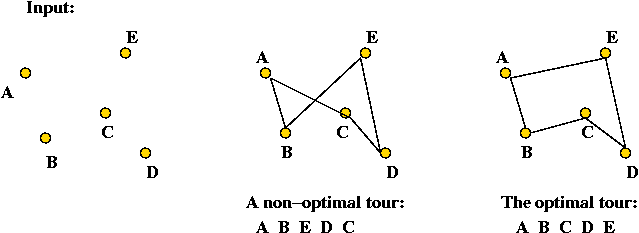• Details:
• Input will be a list of n points, e.g., (x0, y0), (x1, y1), ..., (xn-1, yn-1).
• Solution space: all possible tours.
• "Cost" of a tour: total length of tour.
→ sum of distances between points along tour
• Goal: find the tour with minimal cost (length).

• Strictly speaking, we have defined the Euclidean TSP.

• There are really three kinds:
• The Euclidean (points on the plane).
• The metric TSP: triangle inequality is satisfied.
• The graph TSP: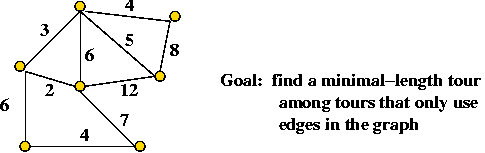Exercise:

• For an n-point problem, what is the size of the solution space (i.e., how many possible tours are there)?
• What's an example of an instance that's metric but not Euclidean?

Some assumptions and notation for the remainder:

• Let n = |V| = number of vertices.
• Euclidean version, unless otherwise stated.
Complete graph.

## Some history

Early history:

• 1832: informal description of problem in German handbook for traveling salesmen.

• 1883 U.S. estimate: 200,000 traveling salesmen on the road

• 1850's onwards: circuit judges

Exercise: Find the following 14 cities in Illinois/Indiana on a map and identify the best tour you can:

Bloomington, Clinton, Danville, Decatur, Metamora, Monticello, Mt.Pulaski, Paris, Pekin, Shelbyville, Springfield, Sullivan, Taylorville, Urbana

• 1960's: Proctor and Gamble \$10K competition: a 33-city TSP.
Won by a CMU mathematician (and others).

• A related problem: the Knight's tour.
Start at bottom-left corner, and visit all squares exactly once and return to the start.

Exercise: Show how the Knight's tour can be converted into a TSP instance.

• The statisticians take an interest
What is the expected length of an optimal tour for uniformly-generated points in 2D?
• Several early analytic estimates in the 1940's.
• Famous Beardwood-Halton-Hammersley result [Bear1959]:
If L* = optimal tour's length then L* / √n   →   a constant β
• β estimated to be 0.72 for unit-square.

• Human solutions:
• To assess problem-solving skill.
• Part of some neurological tests.

TSP's importance in computer science:

• TSP has played a starring role in the development of algorithms.

• Used as a test case for almost every new (discrete) optimization algorithm:
• Branch-and-bound.
• Integer and mixed-integer algorithms.
• Local search algorithms.
• Simulated annealing, Tabu, genetic algorithms.
• DNA computing.

Some milestones:

• Best known optimal algorithm: Held-Karp algorithm in 1962, O(n22n).

• Proof of NP-completeness: Richard Karp in 1972 [Karp1972].
Reduction from Vertex-Cover (which itself reduces from 3-SAT).

• Two directions for algorithm development:
• Faster exact solution approaches (using linear programming).
Largest problem solved optimally: 85,900-city problem (in 2006).
• Effective heuristics.
1,904,711-city problem solved within 0.056% of optimal (in 2009)

• Optimal solutions take a long time
A 7397-city problem took three years of CPU time.

• Theoretical development: (let LH = tour-length produced by heuristic, and let L* be the optimal tour-length)
• 1976: Sahni-Gonzalez result [Sahn1976]. Unless P=NP no polynomial-time TSP heuristic can guarantee LH/L* ≤ 2p(n) for any fixed polynomial p(n).
• Various bounds on particular heuristics (see below).
• 1992: Arora et al result [Aror1992]. Unless P=NP, there exists ε>0 such that no polynomial-time TSP heuristic can guarantee LH/L* ≤ 1+ε for all instances satisfying the triangle inequality.
• 1998: Arora result [Aror1998]. For Euclidean TSP, there is an algorithm that is polyomial for fixed ε>0 such that LH/*H ≤ 1+ε

## Approximate solutions: nearest neighbor algorithm

Nearest-neighbor heuristic:

• Possibly the simplest to implement.

• Sometimes called Greedy in the literature.

• Algorithm:
```
1.   V = {1, ..., n-1}          // Vertices except for 0.
2.   U = {0}                    // Vertex 0.
3.   while V not empty
4.      u = most recently added vertex to U
5.      Find vertex v in V closest to u
6.      Add v to U and remove v from V.
7.   endwhile
8.   Output vertices in the order they were added to U
```

Exercise: What is the solution produced by Nearest-Neighbor for the following 4-point Euclidean TSP. Is it optimal?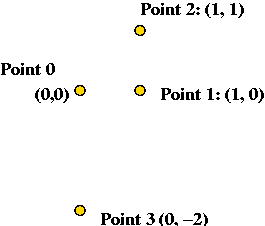• What we know about Nearest-Neighbor:
• LH/L* ≤ O(log n)
• There are instances for which LH/L* = O(log n)
• There are sub-classes of instances for which Nearest-Neighbor consistently produces the worst tour [Guti2007].

## Approximate solutions: the Clarke-Wright heuristic

The Clarke-Wright algorithm: [Clar1964].

• The idea:
• First identify a "hub" vertex: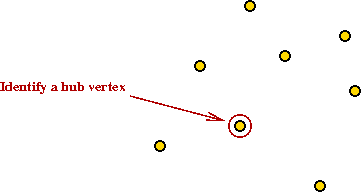• Compute starting cost as cost of going through hub: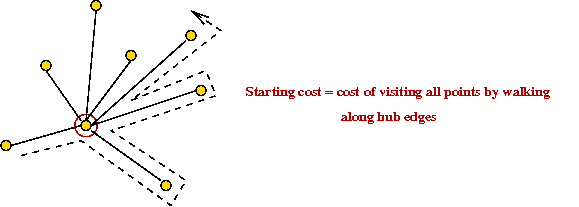• Identify "savings" for each pair of vertices: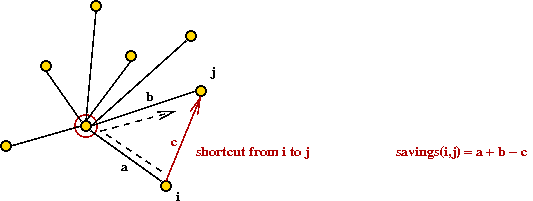• Take shortcuts and add them to final tour, as long as no cycles are created.

• Algorithm:
```
1.   Identify a hub vertex h
2.   VH = V - {h}
3.   for each i,j != h
4.      compute savings(i,j)
5.   endfor
6.   sortlist = Sort vertex pairs in decreasing order of savings
7.   while |VH| > 2
8.      try vertex pair (i,j) in sortlist order
9.      if (i,j) shortcut does not create a cycle
and degree(v) ≤ 2 for all v
10.          add (i,j) segment to partial tour
11.          if degree(i) = 2
12.             VH =  VH - {i}
13.          endif
14.          if degree(j) = 2
15.             VH =  VH - {j}
16.          endif
17.     endif
18.  endwhile
19.  Stitch together remaining two vertices and hub into final tour
```

• Example (from above):
• Suppose vertex 4 is the hub vertex: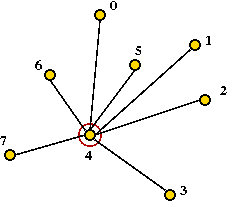• Suppose (2,3) provides the most savings: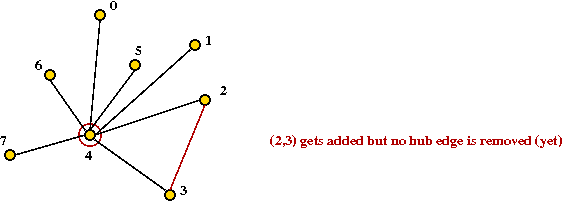degree(2) = 2
must remove hub edge (2,4)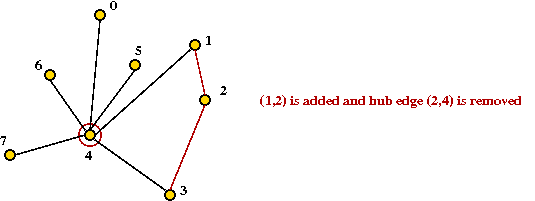• Continuing ... let's say we obtain: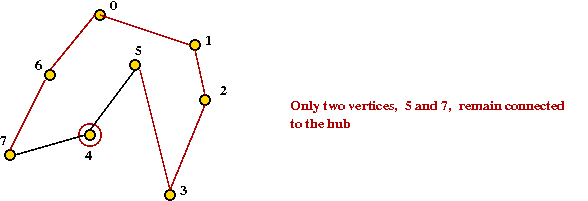• Finally, add last two vertices and hub into final tour: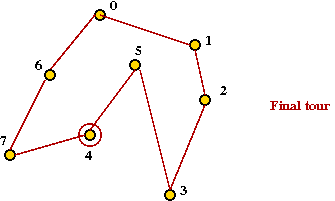• What's known about the CW heuristic:
• Bound is logarithmic: LH/L* ≤ O(log n)
• Worst examples known: LH/L* ≥ O(log(n) / loglog(n))

## Approximate solutions: the MST heuristic

An approximation algorithm for (Euclidean) TSP that uses the MST: [Rose1977].

• The algorithm:
1. First find the minimum spanning tree (using any MST algorithm).
2. Pick any vertex to be the root of the tree.
3. Traverse the tree in pre-order.
4. Return the order of vertices visited in pre-order.

Exercise: What is the pre-order for this tree (starting at A)?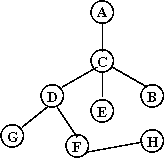• Example:
• Consider these 7 points: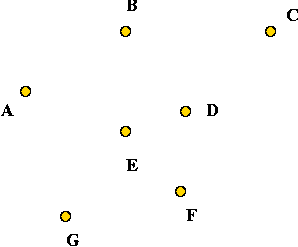• A minimum-spanning tree: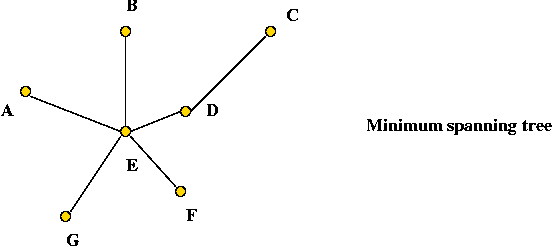• Pick vertex A as the root: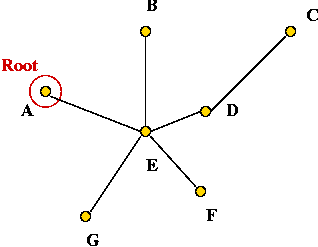• Traverse in pre-order: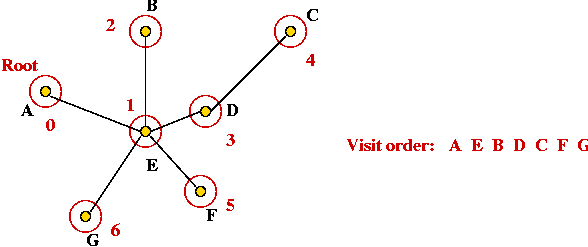• Tour: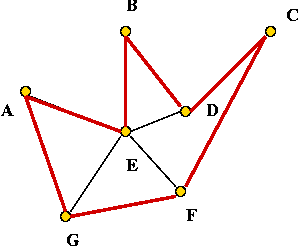• Claim: the tour's length is no worse than twice the optimal tour's length.
• Let L = the length of the tour produced by the algorithm.
• Let L* = the length of an optimal tour.
• Let M = weight of the MST (total length).
• Observe: if we remove any one edge from a tour, we will get a spanning tree.
L* > M.
• Now consider a pre-order tree walk from the root, back to the root: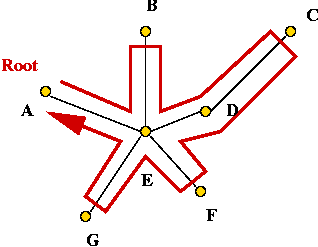• Let W = length of this walk.
• Then, W = 2M (each edge is traversed twice).
• Thus, W < 2L*.
• Finally, we will show that L <= W and therefore, L < 2L*.
• To see why, consider the tree walk from B to D: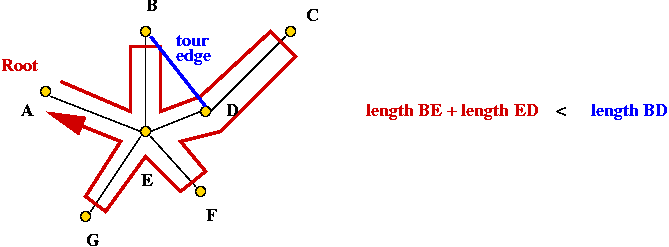→ L takes a shorter route than W (triangle inequality).

• Thus, L <= W.

• The first heuristic to produce solutions within a constant of optimal.
• Easy to implement (since MST can be found efficiently).

## Approximate solutions: the Christofides heuristic

The Christofides algorithm: [Chri1976].

• First, as background, we need to understand two things:
• What is an Euler tour (for general graphs)?
A tour that traverses all edges exactly once (but may repeat vertices)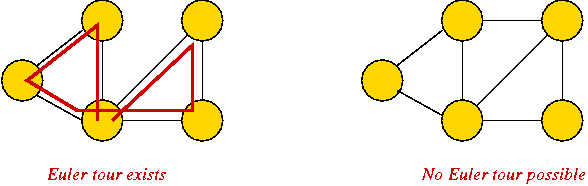• Famous result: a graph has an Euler tour if and only if all its vertices have even degree.
• What is a minimal matching for a given subset of vertices V'?
A "best" (minimal weight) subset of edges with the property that no edges have a common vertex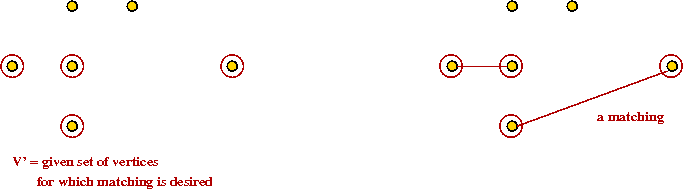• Important result: min-matching can be found in poly-time.

• The key ideas in the algorithm:
• First find the MST• Then identify the odd-degree vertices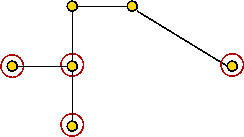• There are an even number of such odd-degree vertices.

Exercise: Why?

• Find a minimal matching of these odd-degree vertices and add those edges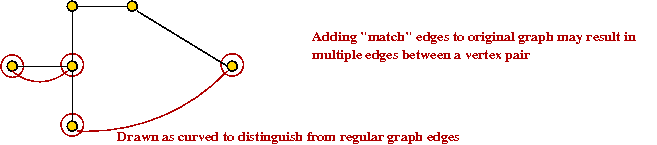• Now all vertices have even degree.

• Next, find an Euler tour.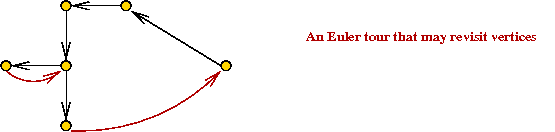• Now, walk along in Euler tour, but skip visited nodes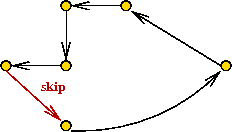• This produces a TSP tour.

An improved bound:

• We will show that LH/L* ≤ 1.5

• Let M = cost of MST.
L* ≥ M (as argued before).

• Note: we are performing a matching on an even number of vertices.

• Now consider the original odd-degree vertices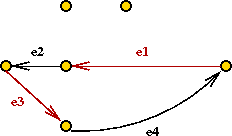• Consider the optimal tour on just these (even # of) vertices.
• Let LO = cost of this tour.
• Let e1, e2, ..., e2k be the edges.
• Note: E1 = {e1, e3, ..., e2k-1} is a matching.
• So is E2 = {e2, e4, ..., e2k}

• Now at least one set has weight at most LO/2.
Because both must add up to LO.

• Also the optimal matching found earlier has less weight than either of these edge sets.
min-match-cost ≤ LO/2 ≤ L*/2.

• Thus min-match-cost + M ≤ L* + L*/2

• But LH uses edges (or shortcuts) from min-match and MST
LH ≤ L* + L*/2

Running time:

• Dominated by O(n3) time for matching.

• Best known matching algorithm: O(n2.376)

## K-OPT

Constructive vs. local-search heuristics:

• All four heuristics above were constructive
A tour was built up step by step.

• In contrast, a local-search heuristic works as follows: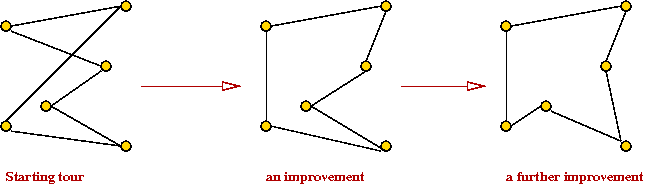```    1.   T = some starting tour      // Perhaps by using Christofides.
2.   noChange = true
3.   repeat
4.      T' = makeChangeTo (T)
5.      if T' < T
6.          T = T'
7.          noChange = false
8.      endif
9.   until noChange
10.  return T
```
2-OPT:
• Idea: Replace 2 edges and see if the cost improves.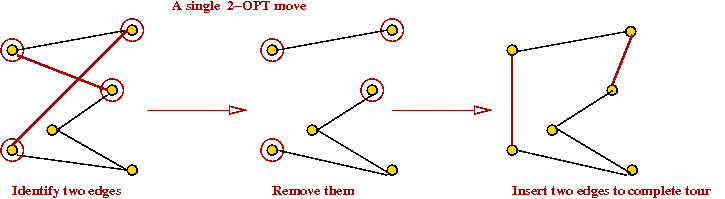• Find two edges and their endpoints.
• Swap endpoints.

• 2-OPT heuristic
```    1.   T = some starting tour
2.   noChange = true
3.   repeat
4.      for all possible edge-pairs in T
5.         T' = tour by swapping end points in edge-pair
6.         if T' < T
7.             T = T'
8.             noChange = false
9.             break      // Quit loop as soon as an improvement is found
10.        endif
11.     endfor
12.  until noChange
13.  return T
```

• An alternative: find best tour with all possible swaps:
```
1.   T = some starting tour
2.   noChange = true
3.   repeat
4.      Tbest = T
5.      for all possible edge-pairs in T
6.         T' = tour by swapping end points in edge-pair
7.         if T' < Tbest
8.             Tbest = T'
9.             noChange = false
10.        endif
11.     endfor
12.     T = Tbest
13.  until noChange
14.  return T
```
K-OPT:
• 3-OPT is what you can get by considering replacing 3 edges.

• K-OPT considers K edges.

• Each K-OPT can be time-consuming for K > 3.
• For general graphs: LH/L* ≤ 0.25 n1/2k.

• For Euclidean case, LH/L* ≤ O(log n).

• In practice: 2-OPT and 3-OPT are much better than the construction heuristics.

• Note: Any K-OPT move can be reduced to a sequence of 2-OPT moves.
But might it might require a long such sequence.

## Local Optima and Problem Landscape

Local optima:

• Recall: greedy-local-search generates one state (tour) after another until no better neighbor can be found
→ does this mean the last one is optimal?

• Observe the trajectory of states: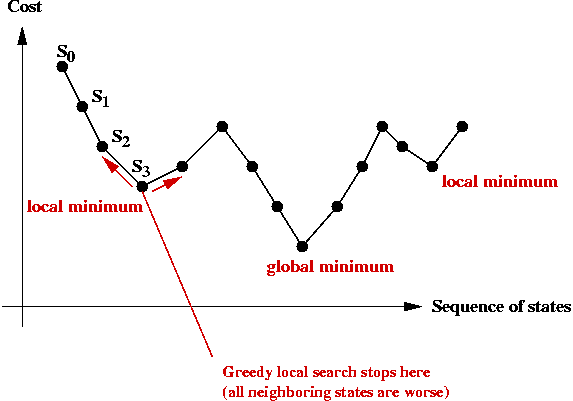• There is no guarantee that a greedy local search can find the (global) minimum.

• The last state found by greedy-local-search is a local minimum.
→ it is the "best" in its neighborhood.

• The global minimum is what we seek: the least-cost solution overall.

• The particular local minimum found by greedy-local-search depends on the start state: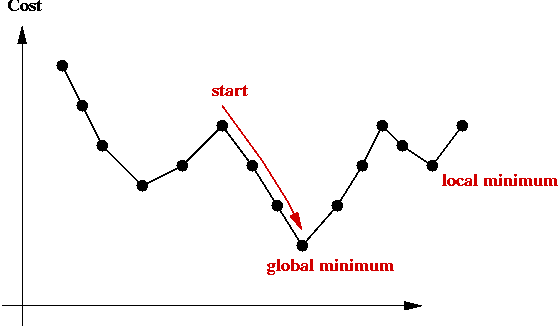Problem landscape:

• Consider TSP using a particular local-search algorithm:
• Suppose we use a graph where the vertices represent states.
• An edge is placed between two "neighbors"
e.g., for a 5-point TSP the neighbors of [0 1 2 3 4] are: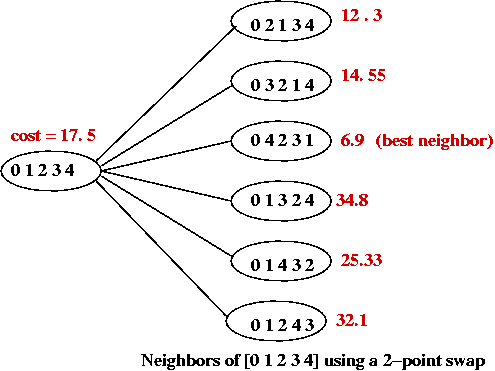• The cost of each tour is represented as the "weight" of each vertex.
• Thus, a local-search algorithm "wanders" around this graph.

• Picture a 3D surface representing the cost above the graph.
→ this is the problem landscape for a particular problem and local-search algorithm.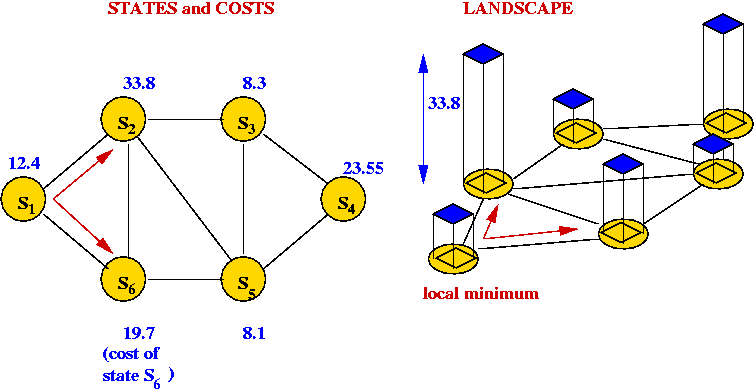• A large part of the difficulty in solving combinatorial optimization problems is the "weirdness" in landscapes
→ landscapes often have very little structure to exploit.

• Unlike continuous optimization problems, local shape in the landscape does NOT help point towards the global minimum.

Climbing out of local minima:

• A local-search algorithm gets "stuck" in a local minimum.

• One approach: re-run local-search many times with different starting points.

• Another approach (next): help a local-search algorithm "climb" out of local minima.

## Tabu search

Key ideas: [Glov1990].

• Suppose we decide to climb out of local minima.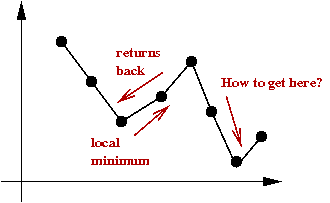• In tabu-search, you maintain a list of "tabu tours".
The algorithm avoids these.

• Each time you pick a minimum in a neighborhood, add that to the tabu list.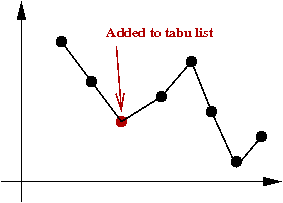• Various alternatives to tabu-lists
• Always add all neighborhood minimums.

• This way, Tabu forces more searching.

• A problem: a tabu-list can grow very long.
Need a policy for removing items, e.g.,
• Least-recently used.
• Throw out high-cost tours.

## Simulated annealing

Background:

• What is annealing?
• Annealing is a metallurgic process for improving the strength of metals.
• Key idea: cool metal slowly during the forging process.

• Example: making bar magnets:
• Wrong way to make a magnet:
1. Heat metal bar to high temperature in magnetic field.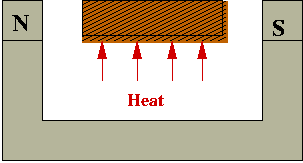2. Cool rapidly (quench):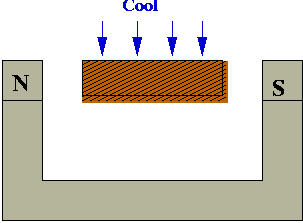• Right way: cool slowly (anneal)

• Why slow-cooling works:
• At high heat, magnetic dipoles are agitated and move around:• The magnetic field tries to force alignment:• If cooled rapidly, alignments tend to be less than optimal (local alignments):• With slow-cooling, alignments are closer to optimal (global alignment):• Summary: slow-cooling helps because it gives molecules more time to "settle" into a globally optimal configuration.

• Relation between "energy" and "optimality"
• The more aligned, the lower the system "energy".
• If the dipoles are not aligned, some dipoles' fields will conflict with others.
• If we (loosely) associate this "wasted" conflicting-fields with energy
→ better alignment is equivalent to lower energy.
• Global minimum = lowest-energy state.

• The Boltzmann Distribution:
• Consider a gas-molecule system (chamber with gas molecules):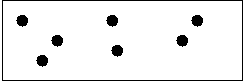• The state of the system is the particular snapshot (positions of molecules) at any time.
• There are high-energy states:and low-energy states:• Suppose the states s1, s2, ... have energies E(s1), E(s2), ...
• A particular energy value E occurs with probability

### P[E] = Z e-E/kT

where Z and k are constants.

• Low-energy states are more probable at low temperatures:
• Consider states s1 and s2 with energies E(s2) > E(s1)
• The ratio of probabilities for these two states is:

### r = P[E(s1)] / P[E(s2)] = e[E(s2) - E(s1)] / kT = exp ([E(s2) - E(s1)] / kT)

Exercise : Consider the ratio of probabilities above:

• Question: what happens to r as T increases to infinity?
• Question: what happens to r as T decreases to zero?
What are the implications?

Key ideas in simulated annealing: [Kirk1983].

• Simulated annealing = a modified local-search.

• Use it to solve a combinatorial optimization problem.

• Associate "energy" with "cost".
→ Goal: find lowest-energy state.

• Recall problem with local-search: gets stuck at local minimum.

• Simulated annealing will allow jumps to higher-cost states.

• If randomly-selected neighbor has lower-cost, jump to it (like local-search does).

• If randomly-selected neighbor is of higher-cost
→ flip a coin to decide whether to jump to higher-cost state
• Suppose current state is s with cost C(s).
• Suppose randomly-selected neighbor is s' with cost C(s') > C(s).

### e -[C(s') - C(s)] / kT

• Decrease coin-flip probability as time goes on:
→ by decreasing temperature T.

• Probability of jumping to higher-cost state depends on cost-difference: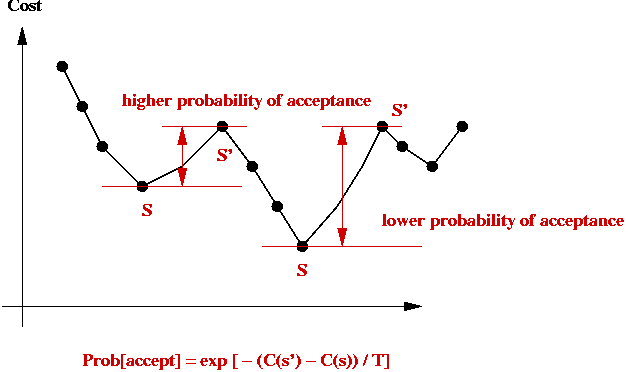Implementation:

• Pseudocode: (for TSP)
```
Algorithm: TSPSimulatedAnnealing (points)
Input: array of points

1.   s = initial tour 0,1,...,n-1

// Record initial tour as best so far.
2.   min = cost (s)
3.   minTour = s

// Pick an initial temperature to allow "mobility"
4.   T = selectInitialTemperature()

// Iterate "long enough"
5.   for i=1 to large-enough-number
// Randomly select a neighboring state.
6.         s' = randomNextState (s)
7.         if cost(s') < cost(s)
8.             s = s'
// Record best so far:
9.             if cost(s') < min
10.                min = cost(s')
11.                minTour = s'
12.            endif
13.        else if expCoinFlip (s, s')
14.            s = s'
15.        endif       // Else stay in current state.
// Decrease temperature.
16.        T = newTemperature (T)
17.  endfor

18.  return minTour

Output: best tour found by algorithm
```
```
Algorithm: randomNextState (s)
Input: a tour s, an array of integers

// ... Swap a random pair of points ...

Output: a tour
```
```
Algorithm: expCoinFlip (s, s')
Input: two states s and s'

1.   p = exp ( -(cost(s') - cost(s)) / T)
2.   u = uniformRandom (0, 1)
3.   if u < p
4.       return true
5.   else
6.       return false

Output: true (if coinFlip resulted in heads) or false
```

• Implementation for other problems, e.g., BPP
• The only thing that needs to change: define a nextState method for each new problem.
• Also, some experimentation will be need for the temperature schedule.

Temperature issues:

• Initial temperature:
• Need to pick an initial temperature that will accept large cost increases (initially).
• One way:
• Guess what the large cost increase might be.
• Pick initial T to make the probability 0.95 (close to 1).

• Decreasing the temperature:
• We need a temperature schedule.
• Several standard approaches:
• Multiplicative decrease: Use T = a * T, where a is a constant like 0.99.
Tn = an.
• Additive decrease: Use T = T - a, where a is a constant like 0.0001.
• Inverse-log decrease: Use T = a / log(n).
• In practice: need to experiment with different temperature schedules for a particular problem.

Analysis:

• How long do we run simulated annealing?
• Typically, if the temperature is becomes very, very small there's no point in further execution
→ because probability of escaping a local minimum is miniscule.

• Unlike previous algorithms, there is no fixed running time.

• What can we say theoretically?
• If the inverse-log schedule is used
→ Can prove "probabilistic convergence to global minimum"
→ Loosely, as the number of iterations increase, the probability of finding the global minimum tends to 1.

In practice:

• Simple to implement.
• Does not need much insight into problem structure.
• Can produce reasonable solutions.
• If greedy does well, so will annealing.

• Poor temperature schedule can prevent sufficient exploration of state space.
• Can require some experimentation before getting it to work well.

• Precautions:
• Always re-run with several (wildly) different starting solutions.
• Always experiment with different temperature schedules.
• Always pick an initial temperature to ensure high probability of accepting a high-cost jump.
• If possible, try different neighborhood functions.

• Warning:
• Just because it has an appealing origin, simulated annealing is not guaranteed to work
→ when it works, it's because it explores more of the state space than a greedy-local-search.
• Simply running greedy-local-search on multiple starting points may be just as effective, and should be experimented with.

Variations:

• Use greedyNextState instead of the nextState function above.
• Advantage: guaranteed to find local minima.
• Disadvantage: may be difficult or impossible to climb out of a particular local minimum:
• Suppose we are stuck at state s, a local minimum.
• When in s', we will very likely jump back to s (unless a better state lies on the "other side").
• Selecting a random next-state is more amenable to exploration.
→ but it may not find local minima easily.

• Hybrid nextState functions:
• Instead of considering the entire neighborhood of 2-swaps, examine some fraction of the neighborhood.
• Switch between different neighborhood functions during iteration.

• Maintain "tabu" lists:
• To avoid jumping to states already seen before, maintain a list of "already-visited" states and exclude these from each neighborhood.

• Thermal cycling:
• Periodically raise temperature and perform "re-starts".
• The idea is to force more exploration of the state space.

## The Held-Karp lower bound

Our presentation will follow the one in [Vale1997].

First, a definition:

• Consider a graph with vertices {1,...,n}: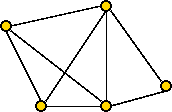• A 1-tree is a subgraph constructed as follows: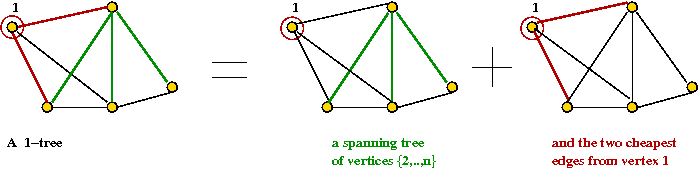• Temporarily remove vertex 1 (and its edges) and find a spanning tree for vertices {2,..,n}.
• Then pick add two cheapest edges from vertex 1.

• Note: every tour (including the optimal one) is a 1-tree.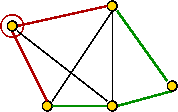• The min-1-tree is the lowest weighted 1-tree among all 1-trees.
This will be a lower bound for the optimal tour.

• A simple algorithm for the min-1-tree:
• Find the MST for the graph without vertex 1.
• Add the two cheapest edges from vertex 1.

• Is the min-1-tree a good bound?

Exercise: What is the difference between the optimal tour and the min-1-tree for this graph?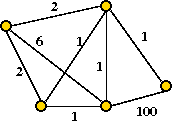• The problem is: the MST can avoid using edges that the tour must take.

Held-Karp's idea:

• We will associate a πi, a vertex weight with every vertex i.

• Define a modifed graph G' as follows:
• G' has the same vertices and edges as G.
• Let eij = weight of edge (i,j) in G.
• Let cij = weight of edge (i,j) in G'.
• Then define cij = eij + πi + πj.

• For example: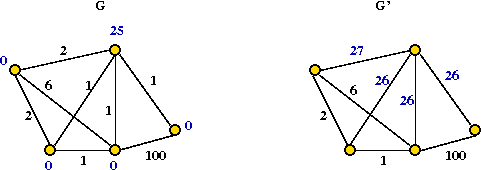Exercise: What is the difference between the min-1-tree and the optimal tour for the above modified graph G'? What vertex weight for the top-right vertex best closes the gap between the min-1-tree and the optimal tour?

• Thus, one can choose weights so that the min-1-tree is as high as possible in G'.

In more detail:

• Let T be a 1-tree and T' be a tour.

• Let dTi = the degree of node i in T.

• Let L(T,G) = cost of 1-tree T using graph G.

• Let L(T',G) = cost of tour T' using graph G.

• Since every tour is a 1-tree, minT L(T,G) ≤ minT' L(T',G).

• Now, for a 1-tree T,
L(T,G') = L(T,G) + ΣiεT (diTi.

• Similarly, for a tour T',
L(T',G') = L(T',G) + ΣiεT'i.

• Thus, subtracting and taking minimum, minT L(T,G) + ΣiεT (diT-2)πi   ≤   minT' L(T',G) = L* (the optimal tour).

• To summarize, we want to find the min-1-tree with weights π and then correct for that by subtracting off the additional weights.

• Let W(π) = minT L(T,G) + ΣiεT (diT-2)πi.

• Then, the desired "best" Held-Karp bound is: maxπ W(π).

An optimization procedure:

• Let VT(π) be the vector (dT1, ..., dTn).

• Let CT(π) be the cost of min-1-tree using π.

• Then, write W(π) = CT(π) + π VT(π).

• Next, suppose that π' is a vector in π-space such that W(π') ≥ W(π).

• Then, Held-Karp show that (π' - π) VT(π) ≥ 0.

• This means that larger values of W(π') are in the right half-space pointed to by the vector VT(π).

• Next step: an iterative optimization procedure.

First, a little background on gradient-based optimization:

• Consider a (single-dimensional) function f(x):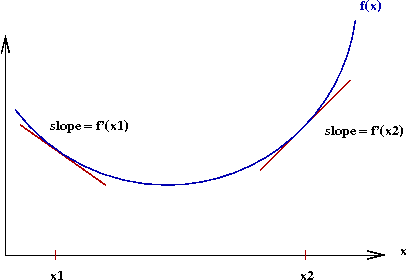• Let f'(x) denote the derivative of f(x).
• The gradient at a point x is the value of f'(x).
→ Graphically, the slope of the tangent to the curve at x.

• Observe the following: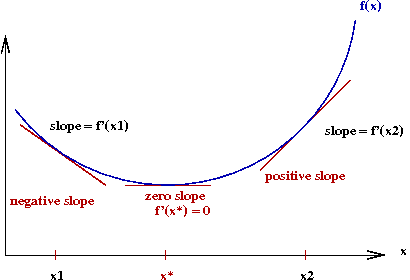• To the left of the optimal value x*, the gradient is negative.
• To the right, it's positive.

• We seek an iterative algorithm of the form
```     while not over
move rightwards
move leftwards
else
stop               // gradient = 0 (unlikely in practice, of course)
endif
endwhile
```

• The gradient descent algorithm is exactly this idea:
```     while not over
x = x - α f'(x)
endwhile
```
Here, we add a scaling factor α in case f'(x) values are of a different order-of-magnitude:

Back to vertex-weight optimization:

• Unfortunately, we don't have a differentiable function.

• For this case, the Russian mathematician Polyak devised what's called the sub-gradient algorithm:
• For a differentiable function, the gradient "points" in the right direction.
• For a non-differentiable function, it's still possible to use a gradient that points in the right direction.

• For the vertex-weights, the iteration turns out to be: πi(m+1)   =   πi(m) + α(m) (di - 2).

• Intuitively, this means:
• Increase the weights for vertices with 1-min-tree degree > 2.
• Decrease the weights for vertices with 1-min-tree degree < 2.
• Thus, the iteration tries to force the 1-min-tree to be "tour-like".

• Polyak showed that sub-gradient iteration works if the stepsizes α(m) are chosen properly:
• α(m)   →   0
• Σm α(m)   =   ∞

• To summarize:
• Repeatedly apply the iteration πi(m+1)   =   πi(m) + stepsize * sub-gradient VT(π).

• Implementation issues:
• Each iteration requires an MST computation.
Can be expensive for large n.
• One approximation: reduce number of edges by considering only best k neighbors (e.g., k=20).

## The Lin-Kernighan algorithm

Key ideas:

• Devised in 1973 by Shen Lin (co-author on BB(N) numbers) and Brian Kernighan (the "K" of K&R fame).

• Champion TSP heuristic 1973-89.

• LK is iterative:
Starts with a tour and repeatedly improves, until no improvement can be found.

• Idea 1: Make the K edges in K-OPT contiguous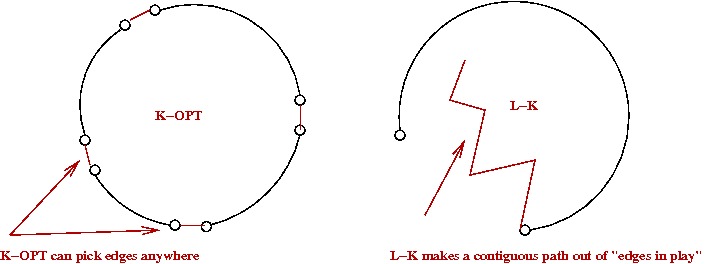• This is just the high-level idea
The algorithm actually alternates between a "current-tour-edge" and a "new-putative-edge".

• Let the K in K-OPT vary at each iteration.
• Try to increase K gradually at each iteration.
• Pick the best K (the best tour) along the way.

• Allow some limited backtracking.

• Use a tabu-list to create freshness in exploration.

Note: we will use an artificial depiction of a tour as follows: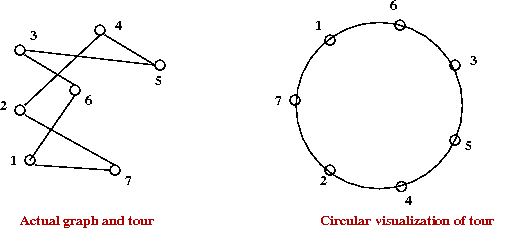This will be used to explain some ideas.

The LK algorithm in more detail:

• At each iteration, LK identifies a sequence of edges x1, y1, x2, y2, ..., xk, yk such that: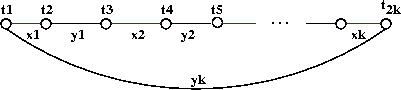• Each xi is an edge in the current tour.
• Each yi is NOT in the current tour.
• They are all unique (no repetitions).
• The last yk returns to the starting point t1

• We'll call this an LK-move.

• For example: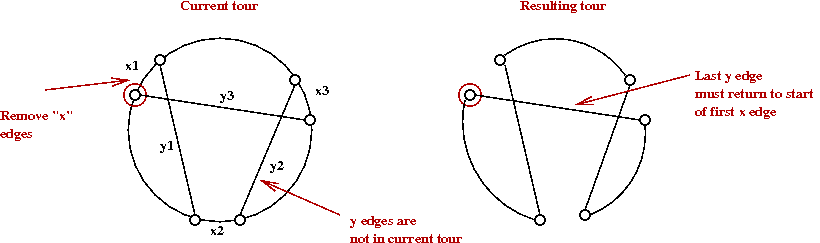• Notice that if we stop at any intermediate yi, we get a 1-tree.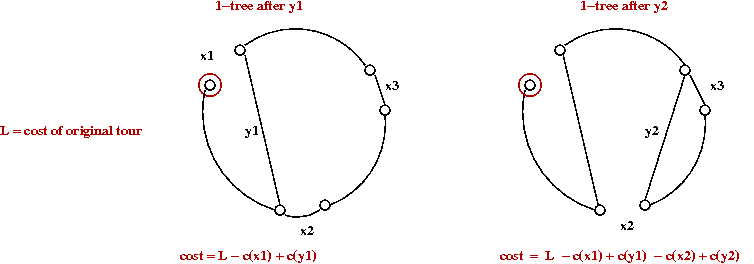• Let G1 = gain after first x-y-pair:
G1 = c(x1) - c(y1)

• Similarly,
G2 = c(x1) - c(y1) + c(x2) - c(y2).

• Gain criterion used by algorithm:
Keep increasing k as long as Gk > 0.

• Note: this is a non-trivial addition because it allows for a temporary loss in gain: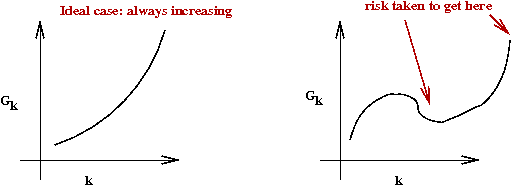• Neighbor limitation: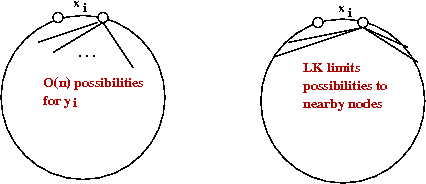• LK limits the number of neighbors to the m nearest neighbors, where m is an algorithm parameter (e.g., m=10).

• Re-starts:
• Recall: there are n choices for t1, the very first node.
• LK tries all n before giving up.

• Best-tour: at all times LK records the best tour found so far.

• Note: LK is actually a little more complicated than described above, but these are the key ideas.

Performance:

• The standard heuristics (construction, K-OPT) give tours with 2-5% above Held-Karp.

• LK is usually between 1-2% off.

## LKH-1: Lin-Kernighan-Helsgaun

From 1999-2009, Keld Helsgaun [Hels2009], added a number of sophisticated optimizations to the basic LK algorithm:

• The first set were added in 1999: [Hels1999].
We'll call this LKH-1.
• And the second set in 2009: [Hels2009].
We'll call this LKH-2.
Key ideas in LKH-1:
• Use K=5 (prefer this value of K over smaller ones).
• Experimental evidence showed that the improvement going from 4- to 5-OPT is much better than 3- to 4-OPT.
• Tradeoff: if K is too high, it takes too long
Fewer iterations
Less exploration of search space (even if you search a particular neighborhood more thoroughly).

• Relax sequentiality allow some xi's and yi's to repeat.

• Replace closest m neighbors with a different set of M neighbors:
• Problem with LK: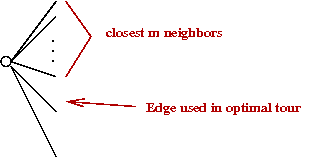• Recall best 1-tree in Held-Karp bound?
Many of these edges are "good" edges for the tour.
Experimental evidence: 70-80% of these edges are in optimal tour.
• LKH-1 idea: prefer 1-tree edges that go to neighbors.
• Let L(T) = cost of best 1-tree
Can be computed fast (MST)
• For any edge e, let
L(T,e) = cost of best 1-tree that must use e.
• How to force using an edge e?
• Find min-1-tree.
• This causes a cycle.
• Remove heaviest edge in cycle.
• This leaves a min-1-tree that uses e.
• Define &alpha(e) = L(T,e) - L(T) = importance of e in "1-tree-ness"
• Note: &alpha(e)=0 for any edge in min-1-tree.
• LKH-1 sorts neighbors by α and uses best m of these.

## LKH-2: Lin-Kernighan-Helsgaun, Part 2

• Allow K to increase beyond 5.

• Problem-partitioning: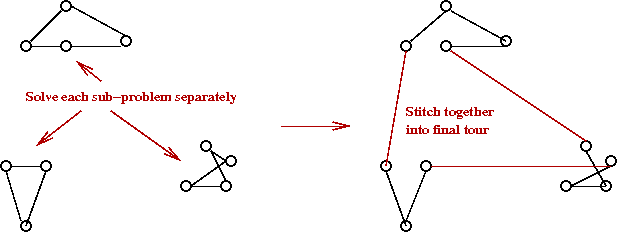• Divide points into clusters.
• Find best tour for each cluster.
• Stitch together into final tour.

• Run algorithm many times and merge "best parts" from multiple tours.
Called iterative partial transcription.

• Use sophisticated tour data structures to speed up running time.

• Results: million city problem with 0.058% of Held-Karp.
Within 0.058% of optimal.

Let's examine the partitioning idea:

• LKH-2 tries a number of partitionings, using different clustering algorithms.

• K-means clustering:
```
1.   repeat
// Note: this is a different K than in K-OPT.
2.      Pick k centroids.
3.      Assign each point to closest centroid.
4.      Re-compute the centroid based on assignments.
5.   until no change
```

• Tour segmentation:
• Run LKH-2 once to find a tour.
• Segment the tour and re-solve the segments (partition).

• Geometric: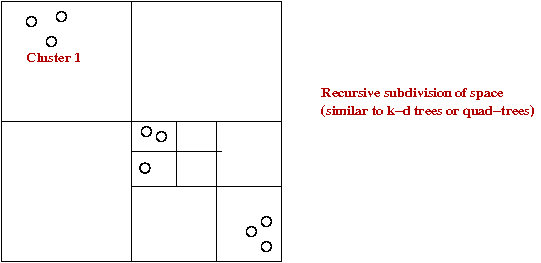• Space-filling curve: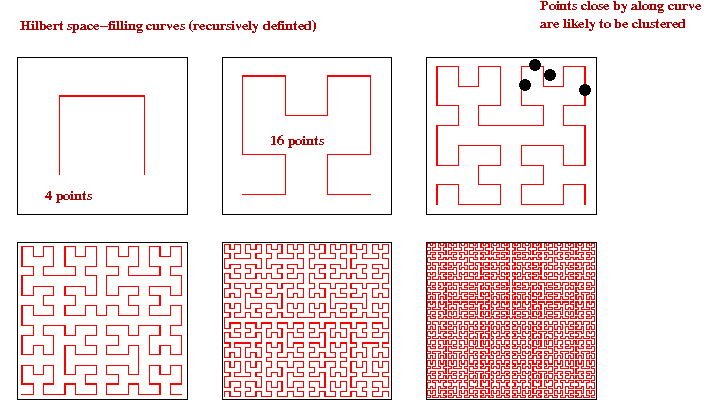Iterative partial transcription (IPT):

• This is an idea from [Mobi1999].

• Goal: given two tours TA and TB, compute TC that is better than both TA and TB.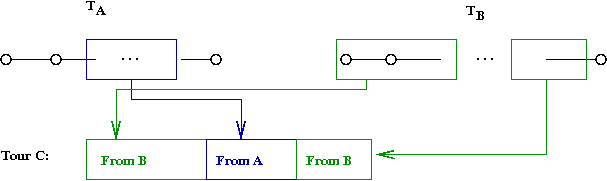• A single IPT trial-swap between tours TA and TB to creates tours TA' and TB'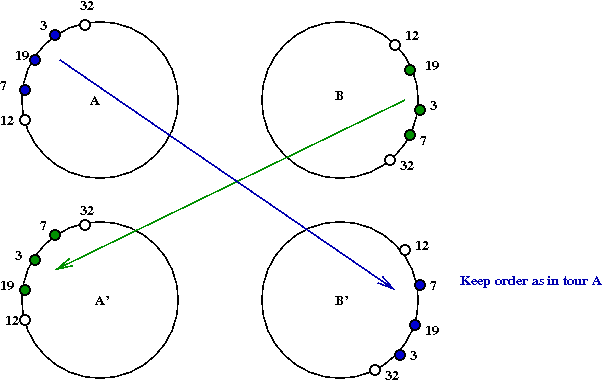• An IPT-iteration:
• Identifies all possible valid swap segments.
• Tries the swaps and identifies the best possible tour that can be generated.

• How to use IPT:
• Generate m tours T1, ..., Tm.
• For each pair of tours i,j, perform an IPT-iteration.

## Data structures

Given a K-OPT move, is the resulting "tour" a valid tour?

• Example: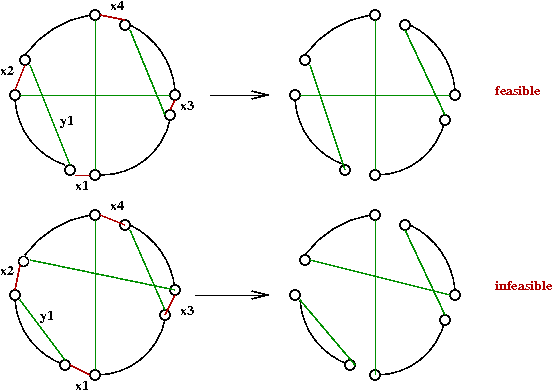• Naive way: walk along new tour T' to see if all vertices are visited
O(n) per trial edge-swap

• Another problem: how to maintain tours?

Operations on tour data structures:

• First, note that any single swap can result in reversing the tour order for one of the segments affected: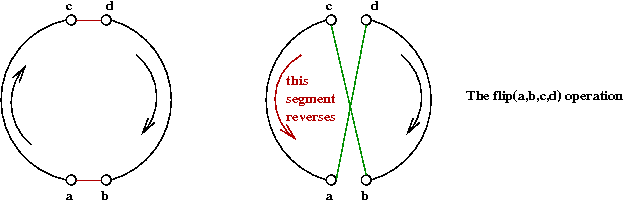• A single 2-OPT move will be called a flip operation.

• Also, any K-OPT move can be implemented by a sequence of 2-OPT moves.
LK-MOVE can be written to use flip operations.

• Other operations that need to be supported:
• next(a): the next node in tour order.
• prev(a): the previous node in tour order.
• between(a,b,c): determine whether b is between a and c in tour-order.

• Note: If a flip is performed correctly, it will result in a valid tour.

• Fredman et al. [Fred1995] show a lower bound of (log n) / (loglog n) for these operations.

Arrays:

• Simple to implement.

• But consider what needs to be done to reverse a segment: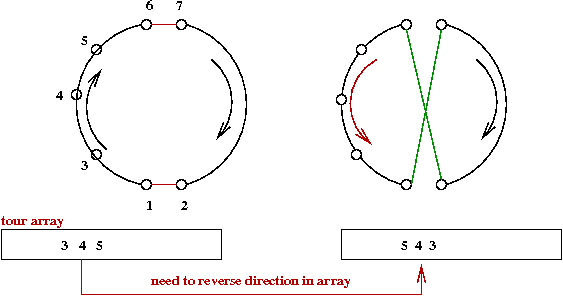Can take O(n).

• flip takes O(1) pointer manipulations.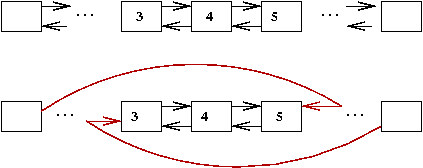• But finding elements is hard: O(n).

Modified splay trees:

• What is a splay tree?
• Also called a self-adjusting binary tree.
• See lecture in algorithms course.
• Recall problem with binary trees: can go out of balance.
• Problem with forced balance (e.g. AVL): too much overhead.
But use of rotations is useful.
• Example of a splay-step: two mini-rotations: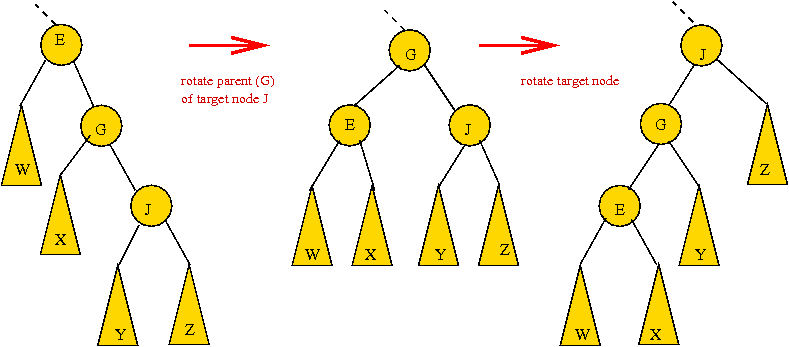• Another example:• In a splay-tree: every accessed node is splayed to the root.
Similar to Move-to-Front in linked lists.

• Using a splay-tree for a tour:
• Each node represents a city.
• Initially, for first tour: in-order traversal is the tour: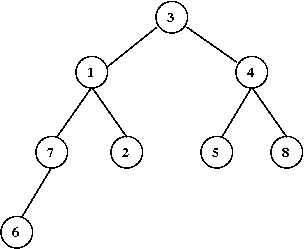Exercise: What is the tour represented by the above tree?

• Reversals are noted by marking intermediate nodes, e.g.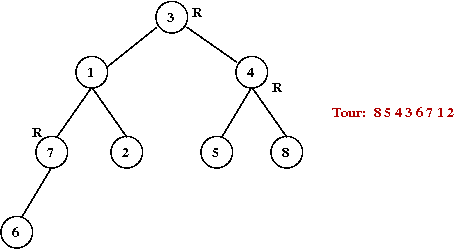• Each time a reversed-node is encountered, switch order (left swapped with right) in in-order traversal: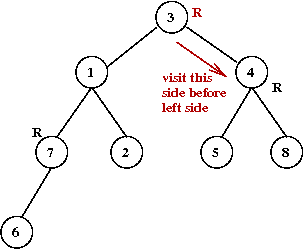• Maintain an external array of pointers into tree, one per node.

• Implementing next(a):
• Recall next(a) in ordinary binary trees: leftmost node of the right subtree.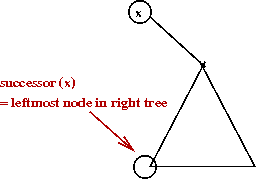• Locate a using pointer-array: O(1).
• Splay to root.
• Find successor using tour-order (instead of numeric order).
With no reversals, this is the leftmost node of the right subtree.
• With reversals, need to change direction for each flip (when recursing).

• The most complex operation is flip():
• Just like the splay-tree, there are several different cases.
• Many involve some type of reversal.
• The general idea (an example):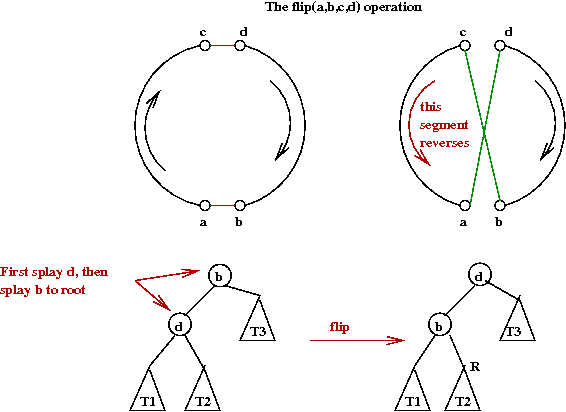The segment tree:

• Devised by Applegate and Cook.

• Based on key observation about LK:
• You try a sequence of flips (the LK-move).
• When it doesn't work, you discard the whole sequence.

• In the data structures so far:
• Every flip changes the data structure.
• To discard, we need to undo flips in reverse order.

• A segment-tree tries to avoid the undo part.
• Array representation of tour.
• An auxiliary segment-list:
To help with tentative flips.
• An auxiliary segment tree:

Performance:

• Segment-tree is usually best.
• 2-level list is next.
• Splay tree next (with theoretically the best performance).

## Exact solution techniques: background

The general idea:

• Formulate TSP as a Integer Programming (IP) problem.

• Apply the cutting-plane approach.

• Judicious choice of cutting-plane heuristics.

But, first, what is Integer Programming? We'll need some background in linear programming.

Linear programming:

• The word program has different meaning than we are used to.
More like a "programme" of events.

• An LP (Linear Programming) problem is (in standard form):
```
max     c1x1 + c2x2 + ... + cnxn
such that
a11x1 + ... +  a1nxn ≤ b1
a21x1 + ... +  a2nxn ≤ b2
.
.
.
an1x1 + ... +  annxn ≤ bn
and
xi ≥ 0,  i=1,...,n
xi ε R
```

• In vector/matrix notation:
```
max   cTx
s.t.  Ax ≤ b
x ≥ 0
```

• Example: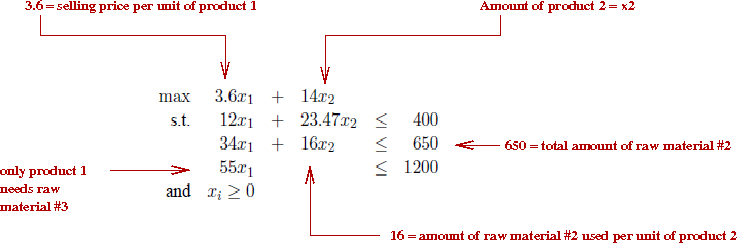• Geometric intuition of inequality constraints (Ax≤b):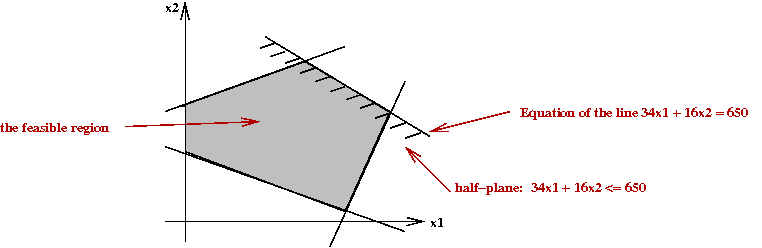• Each inequality defines a half-plane (half-space).
• The intersection is a polytope (polygon in 2D).
• The feasible region is sometimes called the simplex.

• If we plot objective function "lines":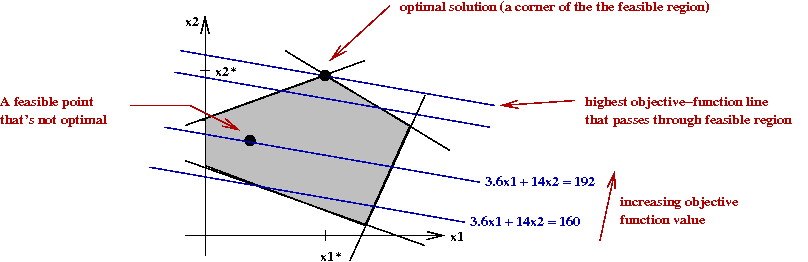• If we make a line-equation out of the objective function, some lines will pass through the feasible region.
• Clearly, we want the line with the highest "value" (for a max problem).
• Sweeping the line upwards (higher value), we want the line that is the last line to intersect the feasible region.
• This line always intersects the region at a corner.

• Three key algorithms, all major milestones in the development of LP:
• George Dantzig's Simplex algorithm (1947).
• Leonid Khachiyan's ellipsoid method (1979).
• Narendra Karmarkar's interior-point method (1984).

• The simplex method: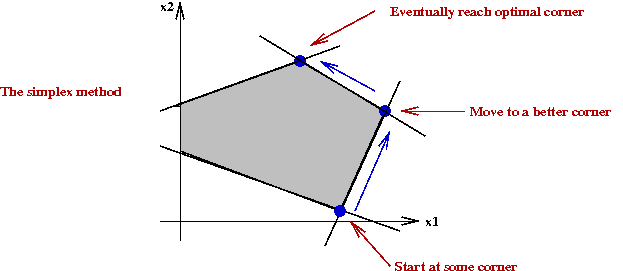• Start at a corner in the feasible region.
• A simplex-move is a move to a neighboring corner.
• Pick a better neighbor to move to (or even best neighbor).
• Repeat until you've reached optimal solution.

• What's known about the simplex method:
• Guaranteed to find optimal solution.
• Worst-case running time: exponential.
• In practice, it's quite efficient, approximately O(n3).
• Very efficient implementations available, both commercial and open-source.
• Has been used to solve very large problems (thousands of variables).

• What's known about the other algorithms:
• Khachiyan's ellipsoid method: provably polynomial, but inefficient in practice.
• Karmarkar's algorithm: provably polynomial and practically efficient for many types of LP problems.

• Note: an LP problem with equality constraints
```
max   cTx
s.t.  Ax = b
x ≥ 0
```
can be converted to an equivalent one in standard form (with inequality constraints).

• Similarly, a min-problem can be convertex to a max-problem.

Integer programming:

• An integer program (IP) is an LP problem with one additional constraint: all xi's are required to be integer:
```
max   cTx
s.t.  Ax ≤ b
x ≥ 0
x ε Z
```

## Exact solution techniques: TSP as an IP problem

First, let's express TSP as an IP problem:

• We'll assume the TSP is a Euclidean TSP (the formulation for a graph-TSP is similar).

• Let the variable xij represent the directed edge (i,j).

• Let cij = cji = the cost of the undirected edge (i,j).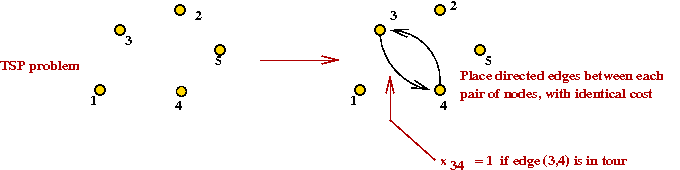• Consider the following IP problem:
```
min    Σi,j  ci,j xi,j
s.t.    Σj xi,j = 1         // Only one outgoing arc from i
Σi xi,j = 1         // Only one incoming arc at j
```

• Unfortunately, this is not sufficient: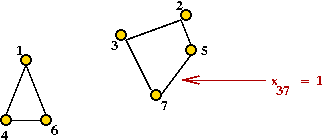You can get multiple cycles.
Called sub-tours

• What to do? Consider this idea: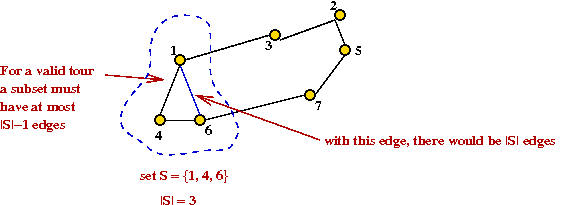• Consider a subset of vertices S.
• In a valid tour,
Σi,j xi,j ≤ |S|-1 for all i,j ε S.
• This is an inequality constraint that could be added to the IP problem.
Called a sub-tour constraint.

• How many such constraints need to be added to the IP problem?
One for each possible subset S.
Exponential number of constraints!

• Fortunately, one can add these constraints only as and when needed (see below).

Solving the IP problem:

• Naive approach:
• Solve the LP relaxation problem first.
Remove integer constraints (temporarily) to get a regular LP, and solve it.
• Round LP solution to nearest integers.
Unfortunately, this may not yield a feasible solution: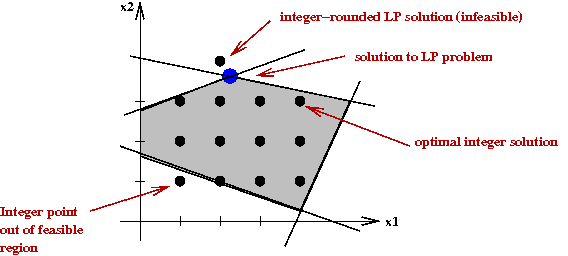• Branch-and-bound:
• We'll explain this for 0-1-IP problems (variables are binary-valued).
• First, consider a simple exhaustive search, organized as a tree-search (the "branch" part):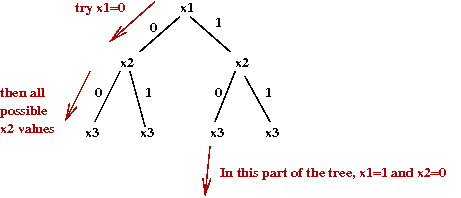• The tree itself can be explored in a variety of ways:
High memory requirements.
• Depth-first
Low memory requirements.
• Cost-first
Expand the node that adds the least overall cost to the (partial) objective function.
• Note: if the cost to a node already exceeds the best tour so far, there's no need to explore further.
Parts of the tree can be pruned.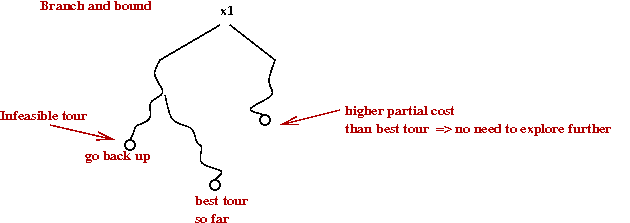• Cutting planes: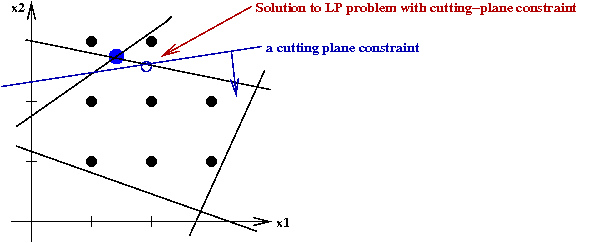• Add constraints to force the LP-solutions towards integers.
• With a sequence of such constraints, such a process can converge to an integer solution.
• However, it can take a long time.

• Gomory's algorithm:
• A general cutting-plane algorithm for any IP.
• The idea:
• Solve LP.
• Examine equations satisfied at corner point (of LP).
• Round to integers in inequalities involving those variables.
• Repeat.
• Unfortunately, it is slow in practice.

History of applying IP to TSP:

• Original cutting plane idea due to Dantzig, Fulkerson and Johnson in 1954.
• Idea:
```
repeat
solve LP
identify sub-tours (cycles) and add corresponding "|S|-1" constraints.
until full-tour found
```
• Dantzig et al added a few more "sub-tour" like constraints.

• Today, there are several families of cutting-plane constraints for the TSP.

• Branch-and-cut
• Cutting planes "ruled" until 1972.
• Saman Hong (JHU) in 1972 combined cutting-planes with branch-and-bound
Called branch-and-cut.
• The idea: some variables might change too slowly with cutting planes
For these, try both 0 and 1 (branch-and-bound idea).
• Alternate way of viewing this: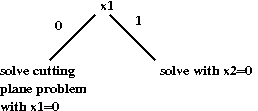• More sophisticated "cut" families:
• Padberg and Hong, 1980: 318-city problem.
• Grotschel and Holland, 1987: 666-city problem.
• Padberg and Rinaldi, 1987-88: combined multiple types of cuts, branch-and-cut and various tricks to solve 2392-city problem.

• During this time, LP techniques improved greatly
Can cut down "active" variables in an LP problem.

• Applegate et al (2006)
• Sophisticated LP techniques, new data structures.
• 85,900 city problem.